# Analysis of the CE amplifier using OrCad Capture.

#### cdummie

Joined Feb 6, 2015
124
Do the bias point analysis, determine voltage gain, find output voltage and find parameter that primarily defines output voltage of the following circuit using simulations in the Orcad Capture.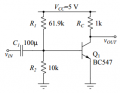Now i'll represent what i have so far:

1) Bias point analysis
This one is quite easy, so i'll just upload image with the final result (i have values of all currents and voltages in the circuit even though we're mainly focused on the values of base-emitter voltage and collector current).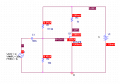I know that i don't need capacitor here since this is "regular" DC analysis so capacitor acts as open circuit and AC input voltage generator acts like short circut). Anyway i have one question here, what VOFF value in AC generator actually represents?

2)Voltage gain
I know that voltage gain represents output voltage divided by input voltage and when we're supposed to solve problems like this manually we simply use small signal model of transistor but here i have to find it different way obviously. So, since i have to represent both input and output signals simply as a functions of time i used Time Domain(Transient) analysis. Since i set the frequency of the input signal to 1kHz it means that one period is equal to 1ms so i set the x axis to 20ms with step size of 0.1ms. Then when i started simulation (after placing voltage markers to input and output) following graphs appeared: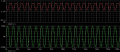Green one (input signal) seems completely fine, but the output is kind of unexpected, i don't understand why it is "displaced". Why it is not oscillating around value of zero volts? I mean is it really positive all the time, and wouldn't it be easier to determine it's amplitude if it was oscillating around zero volts?

3)Output resistance
Output resistance can be found when we add voltage generator to the output and then divide it's voltage with current through it when the input is short circuit.
So basically that is what i did, i adjusted the circuit so it looks something like this: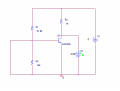Now i had to set the start and end values for V3 generator in DC analysis, since i had values of output voltage in the previous part of this task going from approximately 2.5 to 4.5 volts i set starting value to 2.5V and end value 5V and this is the graph i got: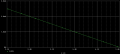I don't know how can i use this diagram to determine output resistance.

4)parameter that primarily defines output voltage
This is the part of the problem that i don't understand at all. What is the parameter that primarily defines output voltage?Is it dissipation power?How am i supposed to determine it's value?

Last edited: